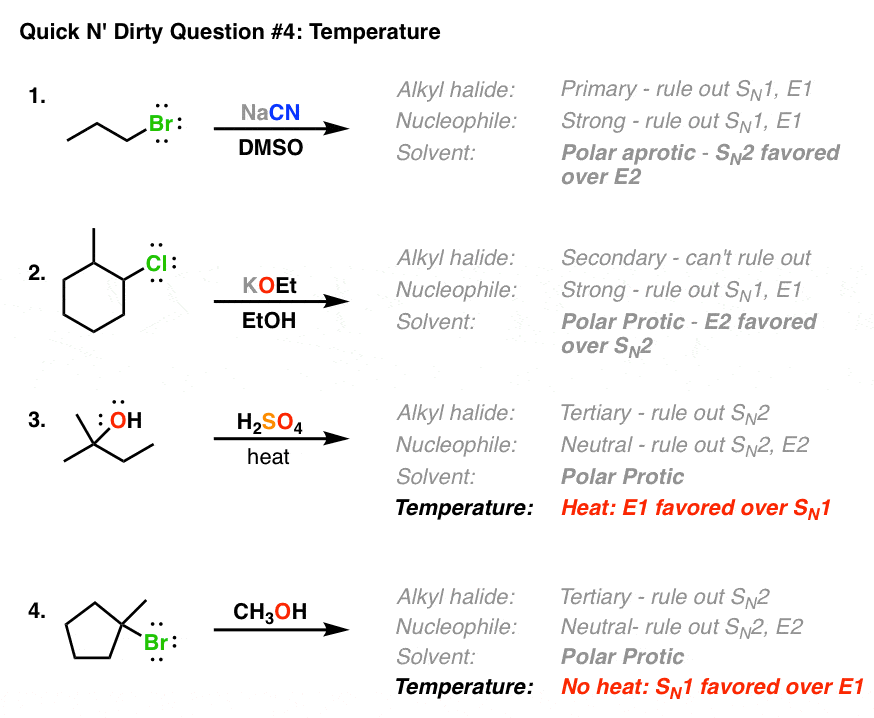# How can I determine whether an E or an SN reaction will occur?

Oct 8, 2015

Four common factors:

• solvent
• sterics
• nucleophile/electrophile strength
(relative to the other)

• temperature

SOLVENT
In Organic Chemistry, you tend to look at:

• Polar Aprotic

EX: Acetone --- Tends to dissolve polar solutes well, and is a hydrogen-bond acceptor. Electrophilic carbonyl carbon.

• Polar Protic

EX: Ethanol --- Tends to dissolve polar solutes well. Can H-bond with reactants because it has a proton. Might deactivate a nucleophile that is also a good base.

• Nonpolar

EX: Alkanes, Benzene, etc.

STERICS
The more steric hindrance you have, the more likely the reaction will be first order. e.g. $E 1$ or ${S}_{N} 1$, depending on the temperature. You might think of this situation as a "passive" substitution or elimination.

The less steric hindrance, the more likely it is second order. e.g. $E 2$ or ${S}_{N} 2$, depending on temperature. You might think of this situation as an "aggressive" substitution or elimination.

NUCLEOPHILE/ELECTROPHILE STRENGTH
The better the nucleophile, the more likely it is ${S}_{N} 2$.

Things like ${H}_{2} N N {H}_{2}$, $C {H}_{3} C {H}_{2} {O}^{-}$, and $O {H}^{-}$ usually make pretty good nucleophiles. Something like $C {H}_{3} C {H}_{2} C {H}_{2} C {H}_{2}^{-} L {i}^{+}$ makes an exceptional nucleophile, almost always. Little sterics, negatively charged.

The worse the nucleophile, the more likely it is ${S}_{N} 1$. If you have lots of steric hindrance, it is even more likely to be ${S}_{N} 1$.

Water, ethanol, and other neutral compounds are usually bad nucleophiles (amines are one of the exceptions). But remember, this is all contextual, so remember your pKas and consider sterics.

TEMPERATURE
The higher the temperature, the more likely elimination occurs.

The lower the temperature, the more likely substitution occurs.

Most effective to control this last, because some substances have low boiling points, flash points, etc. Don't want to lose your reagent(s) or things to explode! You can label heat with $\Delta$ like this:

$\stackrel{\Delta}{\to}$

For example, as-is, a secondary alkyl halide in ethanol will probably do primarily ${S}_{N} 1$, but not predominantly ${S}_{N} 1$. Some ${E}_{1}$ or ${S}_{N} 2$ might occur, but not more than 50% of the time. Likely, little ${E}_{2}$ is possible. This is probably the most difficult case.The polar protic solvent would interact with water, sometimes getting deprotonated, sometimes just getting polarized. This polarization leads the solvent to start SLOWLY approaching the electrophilic center and try to interact with it, eventually switching places. Then water deprotonates the product.

• Using stronger nucleophiles with less steric hindrance would increase the frequency of ${S}_{N} 2$.
• Increasing the steric hindrance and/or the temperature would increase the frequency of ${E}_{1}$ or ${E}_{2}$.
• Switching out the solvent for something polar aprotic would promote ${S}_{N} 2$ because it doesn't interact with water and get deprotonated, thereby decreasing the likelihood of the solvent interacting with the reactant.

If you want to force certain reactions, you could have go-to reactions like these:

${S}_{N} 2$: deprotonated alcohol, the same alcohol as solvent, reacting with a ${1}^{o}$ compound

${S}_{N} 1$: neutral alcohol as solvent, reacting with something ${3}^{o}$, low temperature

${E}_{2}$: tert-butoxide, tert-butanol as solvent, warm~hot temperature

${E}_{1}$: Water, tert-butanol solvent, reacting with something sterically hindered, warm~hot temperature

Other examples:Oct 8, 2015

Watch this video.

#### Explanation:

This video features a practice question on the Mechanism of Nucleophilic Substitutions and Elimination Reactions as SN1 and SN2 or E1 and E2. In this video I explain how to solve questions related to nucleophilic substitutions and elimination reactions by predicting the mechanism and therefore drawing the product(s) and I explain the stereochemistry afterward.

Please refer to the following video on Predicting the Mechanism of Nucleophilic Substitutions Reactions as SN1 or SN2:

Please refer to the following video on Predicting the Mechanism of Elimination Reactions as E1 or E2: